# Algebra

9. Aug 2015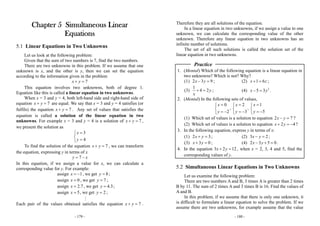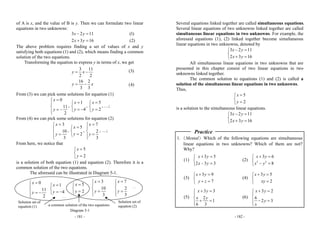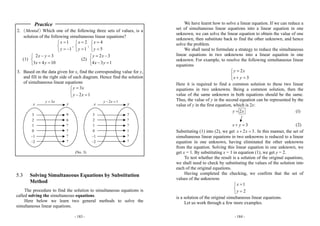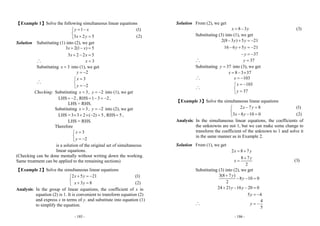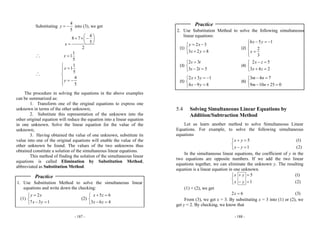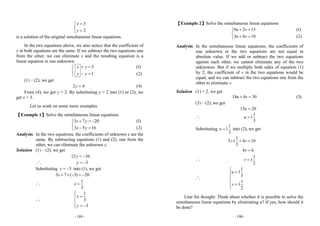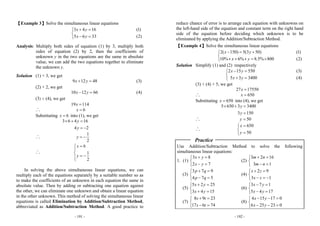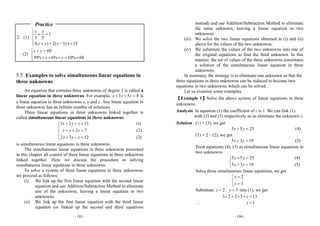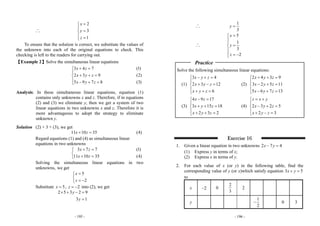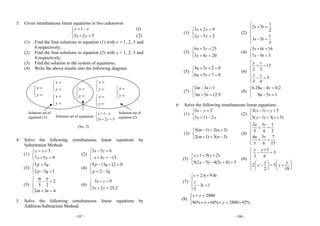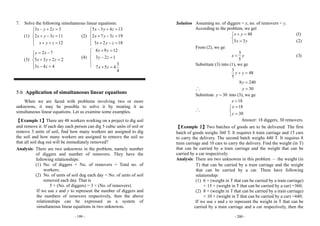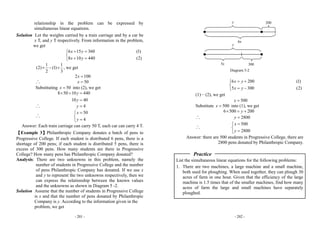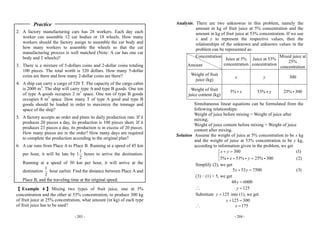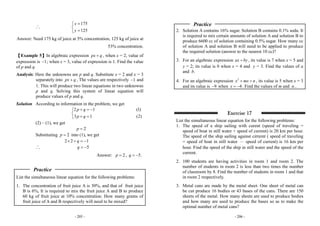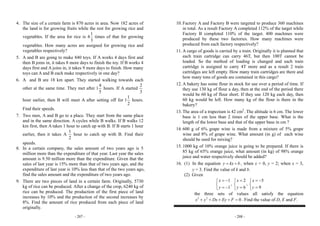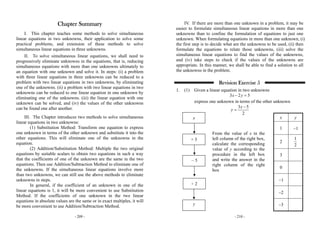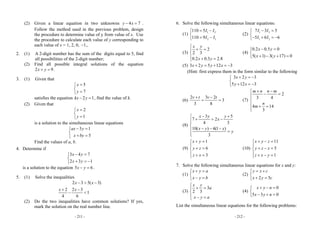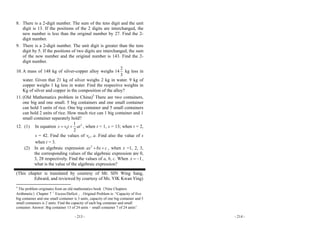1 von 18

### Algebra

• 1. - 179 - Chapter 5 Simultaneous Linear Equations 5.1 Linear Equations in Two Unknowns Let us look at the following problem: Given that the sum of two numbers is 7, find the two numbers. There are two unknowns in this problem. If we assume that one unknown is x, and the other is y, then we can set the equation according to the information given in the problem 7x y+ = This equation involves two unknowns, both of degree 1. Equation like this is called a linear equation in two unknowns. When x = 3 and y = 4, both left-hand side and right-hand side of equation 7x y+ = are equal. We say that x = 3 and y = 4 satisfies (or fulfils) the equation 7x y+ = . Any set of values that satisfies the equation is called a solution of the linear equation in two unknowns. For example x = 3 and y = 4 is a solution of 7x y+ = , we present the solution as 3 4 x y =⎧ ⎨ =⎩ To find the solution of the equation 7x y+ = , we can transform the equation, expressing y in terms of x 7y x= − In this equation, if we assign a value for x, we can calculate a corresponding value for y. For example: assign 1x = − , we get 8y = ; assign 0x = , we get 7y = ; assign 2.7x = , we get 4.3y = ; assign 5x = , we get 2y = ; Each pair of the values obtained satisfies the equation 7x y+ = . - 180 - Therefore they are all solutions of the equation. In a linear equation in two unknowns, if we assign a value to one unknown, we can calculate the corresponding value of the other unknown. Therefore any linear equation in two unknowns has an infinite number of solutions. The set of all such solutions is called the solution set of the linear equation in two unknowns. Practice 1. (Mental) Which of the following equation is a linear equation in two unknowns? Which is not? Why? (1) 2 3 9x y− = ; (2) 1 6x z+ = ; (3) 1 4 2y x + = ; (4) 2 5 3x y− = . 2. (Mental) In the following sets of values, 0 2 x y =⎧ ⎨ = −⎩ , 2 3 x y =⎧ ⎨ = −⎩ , 1 5 x y =⎧ ⎨ = −⎩ (1) Which set of values is a solution to equation 2 7x y− = ? (2) Which set of values is a solution to equation 2 4x y+ = − ? 3. In the following equation, express y in terms of x: (1) 2 3x y+ = ; (2) 3 2x y− = ; (3) 3 0x y+ = ; (4) 2 3 5 0x y− + = . 4. In the equation 3 2 12x y+ = , when x = 2, 3, 4 and 5, find the corresponding values of y. 5.2 Simultaneous Linear Equations in Two Unknowns Let us examine the following problem: There are two numbers A and B, 3 times A is greater than 2 times B by 11. The sum of 2 times A and 3 times B is 16. Find the values of A and B. In this problem, if we assume that there is only one unknown, it is difficult to formulate a linear equation to solve the problem. If we assume there are two unknowns, for example assume that the value
• 2. - 181 - of A is x, and the value of B is y. Then we can formulate two linear equations in two unknowns: 3 2 11 2 3 16 x y x y − = + = (1) (2) The above problem requires finding a set of values of x and y satisfying both equations (1) and (2), which means finding a common solution of the two equations. Transforming the equation to express y in terms of x, we get 3 11 2 2 16 2 3 3 y x y x = − = − (3) (4) From (3) we can pick some solutions for equation (1) 0 11 2 x y =⎧ ⎪ ⎨ = −⎪⎩ , 1 4 x y =⎧ ⎨ = −⎩ , 5 2 x y =⎧ ⎨ =⎩ , …; From (4) we can pick some solutions for equation (2) 3 10 3 x y =⎧ ⎪ ⎨ =⎪⎩ , 5 2 x y =⎧ ⎨ =⎩ , 7 2 3 x y =⎧ ⎪ ⎨ =⎪⎩ , …; From here, we notice that 5 2 x y =⎧ ⎨ =⎩ is a solution of both equation (1) and equation (2). Therefore it is a common solution of the two equations. The aforesaid can be illustrated in Diagram 5-1. a common solution of the two equations Diagram 5-1 Solution set of equation (1) Solution set of equation (2) 0 11 2 x y =⎧ ⎪ ⎨ = −⎪⎩ 1 4 x y =⎧ ⎨ = −⎩ 5 2 x y =⎧ ⎨ =⎩ 3 10 3 x y =⎧ ⎪ ⎨ =⎪⎩ 7 2 3 x y =⎧ ⎪ ⎨ =⎪⎩… … - 182 - Several equations linked together are called simultaneous equations. Several linear equations of two unknowns linked together are called simultaneous linear equations in two unknowns. For example, the aforesaid equations (1), (2) linked together become simultaneous linear equations in two unknowns, denoted by 3 2 11 2 3 16 x y x y − =⎧ ⎨ + =⎩ All simultaneous linear equations in two unknowns that are presented in this chapter consist of two linear equations in two unknowns linked together. The common solution to equations (1) and (2) is called a solution of the simultaneous linear equations in two unknowns. Thus, 5 2 x y =⎧ ⎨ =⎩ is a solution to the simultaneous linear equations. 3 2 11 2 3 16 x y x y − =⎧ ⎨ + =⎩ Practice 1. (Mental) Which of the following equations are simultaneous linear equations in two unknowns? Which of them are not? Why? (1) 3 5 2 3 3 x y x y + =⎧ ⎨ − =⎩ (2) 2 2 3 6 8 x y x y + =⎧ ⎨ − =⎩ (3) 3 9 7 x y y z + =⎧ ⎨ + =⎩ (4) 3 5 2 x y xy + =⎧ ⎨ =⎩ (5) 3 3 2 1 6 3 x y x y + =⎧ ⎪ ⎨ + =⎪⎩ (6) 3 2 6 2 3 x y y x + =⎧ ⎪ ⎨ − =⎪⎩
• 3. - 183 - Practice 2. (Mental) Which one of the following three sets of values, is a solution of the following simultaneous linear equations? 1 1 x y =⎧ ⎨ = −⎩ , 2 1 x y =⎧ ⎨ =⎩ , 4 5 x y =⎧ ⎨ =⎩ (1) 2 3 3 4 10 x y x y − =⎧ ⎨ + =⎩ (2) 2 3 4 3 1 y x x y = −⎧ ⎨ − =⎩ 3. Based on the data given for x, find the corresponding value for y, and fill in the right side of each diagram. Hence find the solution of simultaneous linear equations 3 2 1 y x y x =⎧ ⎨ − =⎩ 5.3 Solving Simultaneous Equations by Substitution Method The procedure to find the solution to simultaneous equations is called solving the simultaneous equations. Here below we learn two general methods to solve the simultaneous linear equations. (No. 3) 3 2 1 0 1− 2− 9 6 ? ? ? ? 3y x= x y 3 2 1 0 1− 2− 7 ? ? 1 ? ? 2 1y x− = x y - 184 - We have learnt how to solve a linear equation. If we can reduce a set of simultaneous linear equations into a linear equation in one unknown, we can solve the linear equation to obtain the value of one unknown, then substitute back to find the other unknown, and hence solve the problem. We shall need to formulate a strategy to reduce the simultaneous linear equations in two unknowns into a linear equation in one unknown. For example, to resolve the following simultaneous linear equations 2 3 y x x y =⎧ ⎨ + =⎩ Here it is required to find a common solution to these two linear equations in two unknowns. Being a common solution, then the value of the same unknown in both equations should be the same. Thus, the value of y in the second equation can be represented by the value of y in the first equation, which is 2x: Substituting (1) into (2), we get 2 3x x+ = . In this manner, the set of simultaneous linear equations in two unknowns is reduced to a linear equation in one unknown, having eliminated the other unknowns from the equation. Solving this linear equation in one unknown, we get x = 1. By substituting x = 1 in equation (1), we get y = 2. To test whether the result is a solution of the original equations, we shall need to check by substituting the values of the solution into each of the original equations. Having completed the checking, we confirm that the set of values of the unknowns 1 2 x y =⎧ ⎨ =⎩ is a solution of the original simultaneous linear equations. Let us work through a few more examples. 2 3 y x x y = + = (1) (2)
• 4. - 185 - 【Example 1】Solve the following simultaneous linear equations 1 3 2 5 y x x y = −⎧ ⎨ + =⎩ (1) (2) Solution Substituting (1) into (2), we get 3 2(1 ) 5 3 2 2 5 x x x x + − = + − = ∴ 3x = Substituting 3x = into (1), we get 2y = − ∴ 3 2 x y =⎧ ⎨ = −⎩ Checking: Substituting 3x = , 2y = − into (1), we get LHS 2= − , RHS 1 3 2= − = − , LHS = RHS. Substituting 3x = , 2y = − into (2), we get LHS =3 3 2 ( 2) 5× + × − = , RHS 5= , LHS = RHS. Therefore 3 2 x y =⎧ ⎨ = −⎩ is a solution of the original set of simultaneous linear equations. (Checking can be done mentally without writing down the working. Same treatment can be applied to the remaining sections) 【Example 2】Solve the simultaneous linear equations 2 5 21 3 8 x y x y + = −⎧ ⎨ + =⎩ (1) (2) Analysis: In the group of linear equations, the coefficient of x in equation (2) is 1. It is convenient to transform equation (2) and express x in terms of y. and substitute into equation (1) to simplify the equation. - 186 - Solution From (2), we get 8 3x y= − (3) Substituting (3) into (1), we get 2(8 3 ) 5 21 16 6 5 21 37 y y y y y − + = − − + = − − = − ∴ 37y = Substituting 37y = into (3), we get 8 3 37x = − × ∴ 103x = − ∴ 103 37 x y = −⎧ ⎨ =⎩ 【Example 3】Solve the simultaneous linear equations 2 7 8 3 8 10 0 x y x y − =⎧ ⎨ − − =⎩ (1) (2) Analysis: In the simultaneous linear equations, the coefficients of the unknowns are not 1, but we can make some change to transform the coefficient of the unknown to 1 and solve it in the same manner as in Example 2. Solution From (1), we get 2 8 7 8 7 2 x y y x = + + = (3) Substituting (3) into (2), we get 3(8 7 ) 8 10 0 2 24 21 16 20 0 5 4 y y y y y + − − = + − − = = − ∴ 4 5 y = −
• 5. - 187 - Substituting 4 5 y = − into (3), we get 4 8 7 5 2 x ⎛ ⎞+ × −⎜ ⎟ ⎝ ⎠= ∴ 1 1 5 x = ∴ 1 1 5 4 5 x y ⎧ =⎪⎪ ⎨ ⎪ = − ⎪⎩ The procedure in solving the equations in the above examples can be summarized as: 1. Transform one of the original equations to express one unknown in terms of the other unknown; 2. Substitute this representation of the unknown into the other original equation will reduce the equation into a linear equation in one unknown. Solve the linear equation for the value of the unknown; 3. Having obtained the value of one unknown, substitute its value into one of the original equations will enable the value of the other unknown be found. The values of the two unknowns thus obtained constitute a solution of the simultaneous linear equations. This method of finding the solution of the simultaneous linear equations is called Elimination by Substitution Method, abbreviated as Substitution Method. Practice 1. Use Substitution Method to solve the simultaneous linear equations and write down the checking: (1) 2 7 3 1 y x x y =⎧ ⎨ − =⎩ (2) 5 6 3 6 4 x z x z + =⎧ ⎨ − =⎩ - 188 - Practice 2. Use Substitution Method to solve the following simultaneous linear equations:    (1)  2 3 3 2 8 y x x y = −⎧ ⎨ + =⎩   (2)  6 5 1 2 3 x y x − = −⎧ ⎪ ⎨ =⎪⎩      (3)  2 3 3 2 5 s t s t =⎧ ⎨ − =⎩   (4)  2 5 3 4 2 x z x z − =⎧ ⎨ + =⎩      (5)  2 3 1 4 9 8 x y x y + = −⎧ ⎨ − =⎩   (6)  3 4 7 9 10 25 0 m n m n − =⎧ ⎨ − + =⎩   5.4 Solving Simultaneous Linear Equations by Addition/Subtraction Method Let us learn another method to solve Simultaneous Linear Equations. For example, to solve the following simultaneous equations 5 1 x y x y + =⎧ ⎨ − =⎩ (1) (2) In the simultaneous linear equations, the coefficient of y in the two equations are opposite numbers. If we add the two linear equations together, we can eliminate the unknown y. The resulting equation is a linear equation in one unknown. 5 1 x y x y + =⎧ ⎨ − =⎩ (1) (2) (1) + (2), we get 2 6x = (3) From (3), we get x = 3. By substituting x = 3 into (1) or (2), we get y = 2. By checking, we know that
• 6. - 189 - 3 2 x y =⎧ ⎨ =⎩ is a solution of the original simultaneous linear equations. In the two equations above, we also notice that the coefficient of x in both equations are the same. If we subtract the two equations one from the other, we can eliminate x and the resulting equation is a linear equation in one unknown. 5 1 x y x y + =⎧ ⎨ − =⎩ (1) (2) (1)－(2), we get 2 4y = (4) From (4), we get y = 2. By substituting y = 2 into (1) or (2), we get x = 3. Let us work on some more examples. 【Example 1】Solve the simultaneous linear equations 3 7 20 3 5 16 x y x y + = −⎧ ⎨ − =⎩ (1) (2) Analysis: In the two equations, the coefficients of unknown x are the same. By subtracting equations (1) and (2), one from the other, we can eliminate the unknown x. Solution (1)－(2), we get 12 36y = − ∴ 3y = − Substituting 3y = − into (1), we get 3 7 ( 3) 20x + × − = − ∴ 1 3 x = ∴ 1 3 3 x y ⎧ =⎪ ⎨ ⎪ = −⎩ - 190 - 【Example 2】Solve the simultaneous linear equations 9 2 15 3 4 10 u v u v + =⎧ ⎨ + =⎩ (1) (2) Analysis: In the simultaneous linear equations, the coefficients of one unknown in the two equations are not equal in absolute value. If we add or subtract the two equations against each other, we cannot eliminate any of the two unknowns. But if we multiply both sides of equation (1) by 2, the coefficient of v in the two equations would be equal, and we can subtract the two equations one from the other to eliminate v. Solution (1) × 2, we get 18 4 30u v+ = (3) (3)－(2), we get 15 20u = ∴ 1 1 3 u = Substituting 1 1 3 u = into (2), we get 1 3 1 4 10 3 4 6 v v × + = = ∴ 1 1 2 v = ∴ 1 1 3 1 1 2 u v ⎧ =⎪⎪ ⎨ ⎪ = ⎪⎩ Line for thought: Think about whether it is possible to solve the simultaneous linear equations by eliminating u? If yes, how should it be done?
• 7. - 191 - 【Example 3】Solve the simultaneous linear equations 3 4 16 5 6 33 x y x y + =⎧ ⎨ − =⎩ (1) (2) Analysis: Multiply both sides of equation (1) by 3, multiply both sides of equation (2) by 2, then the coefficients of unknown y in the two equations are the same in absolute value, we can add the two equations together to eliminate the unknown y. Solution (1) × 3, we get 9 12 48x y+ = (3) (2) × 2, we get 10 12 66x y− = (4) (3) + (4), we get 19 114x = ∴ 6x = Substituting 6x = into (1), we get 3 6 4 16 4 2 y y × + = = − ∴ 1 2 y = − ∴ 6 1 2 x y =⎧ ⎪ ⎨ = −⎪⎩ In solving the above simultaneous linear equations, we can multiply each of the equations separately by a suitable number so as to make the coefficients of an unknown in each equation the same in absolute value. Then by adding or subtracting one equation against the other, we can eliminate one unknown and obtain a linear equation in the other unknown. This method of solving the simultaneous linear equations is called Elimination by Addition/Subtraction Method, abbreviated as Addition/Subtraction Method. A good practice to - 192 - reduce chance of error is to arrange each equation with unknowns on the left-hand side of the equation and constant term on the right hand side of the equation before deciding which unknown is to be eliminated by applying the Addition/Subtraction Method. 【Example 4】Solve the simultaneous linear equations 2( 150) 5(3 50) 10% 6% 8.5% 800 x y x y − = +⎧ ⎨ + = ×⎩ i i (1) (2) Solution Simplify (1) and (2) respectively 2 15 550 5 3 3400 x y x y − =⎧ ⎨ + =⎩ (3) (4) (3) + (4) × 5, we get 27 17550x = ∴ 650x = Substituting 650x = into (4), we get 5 650 3 3400 3 150 y y × + = = ∴ 50y = ∴ 650 50 x y =⎧ ⎨ =⎩ Practice Use Addition/Subtraction Method to solve the following simultaneous linear equations: 1. (1) 3 8 2 7 x y x y + =⎧ ⎨ − =⎩ (2) 3 2 16 3 1 m n m n + =⎧ ⎨ − =⎩ (3) 3 7 9 4 7 5 p q p q + =⎧ ⎨ − =⎩ (4) 2 9 3 1 x z x z + =⎧ ⎨ − = −⎩ (5) 5 2 25 3 4 15 x y x y + =⎧ ⎨ + =⎩ (6) 3 7 1 5 4 17 x y x y − =⎧ ⎨ − =⎩ (7) 8 9 23 17 6 74 s t s t + =⎧ ⎨ − =⎩ (8) 4 15 17 0 6 25 23 0 x y x y − − =⎧ ⎨ − − =⎩
• 8. - 193 - Practice 2. (1) 1 3 5 3( ) 2( 3 ) 15 x y x y x y ⎧ + =⎪ ⎨ ⎪ + + − =⎩ (2) 60 30% 6% 10% 60 x y x y + =⎧ ⎨ + = ×⎩ i i 5.5 Examples to solve simultaneous linear equations in three unknowns An equation that contains three unknowns of degree 1 is called a linear equation in three unknowns. For example,  3 5 4x y z+ + = is a linear equation in three unknowns x, y and z. Any linear equation in three unknowns has an infinite number of solutions. Three linear equations in three unknowns linked together is called simultaneous linear equations in three unknowns. 3 2 13 2 7 2 3 12 x y z x y z x y z + + =⎧ ⎪ + + =⎨ ⎪ + − =⎩ (1) (2) (3) is simultaneous linear equations in three unknowns. The simultaneous linear equations in three unknowns presented in this chapter all consist of three linear equations in three unknowns linked together. Here we discuss the procedure in solving simultaneous linear equations in three unknowns. To solve a system of three linear equations in three unknowns, we proceed as follows: (i) We link up the first linear equation with the second linear equation and use Addition/Subtraction Method to eliminate one of the unknowns, leaving a linear equation in two unknowns. (ii) We link up the first linear equation with the third linear equation (or linked up the second and third equations - 194 - instead) and use Addition/Subtraction Method to eliminate the same unknown, leaving a linear equation in two unknowns. (iii) We solve the two linear equations obtained in (i) and (ii) above for the values of the two unknowns. (iv) We substitute the values of the two unknowns into one of the original equations to find the third unknown. In this manner, the set of values of the three unknowns constitutes a solution of the simultaneous linear equation in three unknowns. In summary, the strategy is to eliminate one unknown so that the three equations in three unknowns can be reduced to become two equations in two unknowns, which can be solved. Let us examine some examples. 【Example 1】Solve the above system of linear equations in three unknowns. Analysis: In equation (1) the coefficient of z is 1. We can link (1) with (2) and (3) respectively so as eliminate the unknown z. Solution (1) + (3), we get 5 5 25x y+ = (4) (1) × 2－(2), we get 5 3 19x y+ = (5) Treat equations (4), (5) as simultaneous linear equations in two unknowns 5 5 25 5 3 19 x y x y + =⎧ ⎨ + =⎩                 (4) (5) Solve these simultaneous linear equations, we get 2 3 x y =⎧ ⎨ =⎩ Substitute 2x = , 3y = into (1), we get 3 2 2 3 13z× + × + = ∴ 1z =
• 9. - 195 - ∴ 2 3 1 x y z =⎧ ⎪ =⎨ ⎪ =⎩ To ensure that the solution is correct, we substitute the values of the unknown into each of the original equations to check. This checking is left to the readers for carrying out. 【Example 2】Solve the simultaneous linear equations 3 4 7 2 3 9 5 9 7 8 x z x y z x y z + =⎧ ⎪ + + =⎨ ⎪ − + =⎩ (1) (2) (3) Analysis: In these simultaneous linear equations, equation (1) contains only unknowns x and z. Therefore, if in equations (2) and (3) we eliminate y, then we get a system of two linear equations in two unknowns x and z. Therefore it is more advantageous to adopt the strategy to eliminate unknown y. Solution (2) × 3 + (3), we get 11 10 35x z+ = (4) Regard equations (1) and (4) as simultaneous linear equations in two unknowns 3 7 7 11 10 35 x z x z + =⎧ ⎨ + =⎩ (1) (4) Solving the simultaneous linear equations in two unknowns, we get 5 2 x z =⎧ ⎨ = −⎩ Substitute 5x = , 2z = − into (2), we get 2 5 3 2 9 3 1 y y × + − = = - 196 - ∴ 1 3 y = ∴ 5 1 3 2 x y z =⎧ ⎪ ⎪ =⎨ ⎪ = −⎪⎩ Practice Solve the following simultaneous linear equations: (1) 3 4 2 3 12 6 x y z x y z x y z − + =⎧ ⎪ + − =⎨ ⎪ + + =⎩ (2) 2 4 3 9 3 2 5 11 5 6 7 13 x y z x y z x y z + + =⎧ ⎪ − + =⎨ ⎪ − + =⎩ (3) 4 9 17 3 15 18 2 3 2 x z x y z x y z − =⎧ ⎪ + + =⎨ ⎪ + + =⎩ (4) 2 3 2 5 2 3 z x y x y z x y z = +⎧ ⎪ − + =⎨ ⎪ + − =⎩ Exercise 16 1. Given a linear equation in two unknowns 2 7 4x y− = (1) Express y in terms of x; (2) Express x in terms of y. 2. For each value of x (or y) in the following table, find the corresponding value of y (or x)which satisfy equation 3 5x y+ = to x 2− 0 2 3 2 y 1 2 − 0 3
• 10. - 197 - 3. Given simultaneous linear equations in two unknowns 1 3 2 5 y x x y = −⎧ ⎨ + =⎩ (1) (2) (1) Find the four solutions to equation (1) with x = 1, 2, 3 and 4 respectively; (2) Find the four solutions to equation (2) with x = 1, 2, 3 and 4 respectively; (3) Find the solution to the system of equations; (4) Write the above results into the following diagram. 4. Solve the following simultaneous linear equations by Substitution Method: (1) 3 7 5 9 y x x y = +⎧ ⎨ + =⎩ (2) 3 5 6 4 15 x z x z − =⎧ ⎨ + = −⎩ (3) 3 5 2 3 1 p q p q =⎧ ⎨ − =⎩ (4) 9 13 12 0 2 3 p q p q − + =⎧ ⎨ = −⎩ (5) 2 5 2 2 3 4 m n m n ⎧ − =⎪ ⎨ ⎪ + =⎩ (6) 3 5 5 2 25.2 x z x z − =⎧ ⎨ + =⎩ 5. Solve the following simultaneous linear equations by Addition/Subtraction Method: (No. 3) Solution set of equation (1) Solution set of equation (2) x y =⎧ ⎨ =⎩ x y =⎧ ⎨ =⎩ x y =⎧ ⎨ =⎩ x y =⎧ ⎨ =⎩ x y =⎧ ⎨ =⎩ Solution set of equations 1 3 2 5 y x x y = −⎧ ⎨ + =⎩ x y =⎧ ⎨ =⎩ x y =⎧ ⎨ =⎩ - 198 - (1) 3 2 9 3 5 2 x y x y + =⎧ ⎨ − =⎩ (2) 1 2 5 2 1 3 5 3 s t s t ⎧ + =⎪⎪ ⎨ ⎪ − = ⎪⎩ (3) 6 5 25 3 4 20 x z x z + =⎧ ⎨ + =⎩ (4) 5 6 16 7 9 5 s t s t + =⎧ ⎨ − =⎩ (5) 8 3 2 0 6 5 7 0 u v u v + + =⎧ ⎨ + + =⎩ (6) 13 2 3 3 3 4 y z y z ⎧ + =⎪⎪ ⎨ ⎪ − = ⎪⎩ (7) 2 3 1 3 5 12.9 m n m n − =⎧ ⎨ + =⎩ (8) 6.28 4 0.2 8 5 1 u v u v − =⎧ ⎨ − =⎩ 6. Solve the following simultaneous linear equations: (1) 3 2 3 11 2 x y x y − =⎧ ⎨ = −⎩ (2) 3( 1) 5 5( 1) 3( 5) x y y x − = +⎧ ⎨ − = +⎩ (3) 5( 1) 2( 3) 2( 1) 3( 3) m n m n − = +⎧ ⎨ + = −⎩ (4) 2 3 1 3 4 2 4 5 7 5 6 15 u v u v ⎧ + =⎪⎪ ⎨ ⎪ + = ⎪⎩ (5) 1 5( 2) 3(2 5) 4(3 4) 5 x z x z + = +⎧ ⎨ − − + =⎩ (6) 1 3 3 6 2 3 182 y x yy xx +⎧ − =⎪⎪ ⎨ ⎛ ⎞⎛ ⎞⎪ = +−⎜ ⎟ ⎜ ⎟ ⎪ ⎝ ⎠ ⎝ ⎠⎩ (7) 2.6 9.8 3 1 3 v t v t = +⎧ ⎪ ⎨ − =⎪⎩ (8) 2800 96% 64% 2800 92% x y x y + =⎧ ⎨ + = ×⎩ i i
• 11. - 199 - 7. Solve the following simultaneous linear equations: (1) 3 2 3 2 3 11 12 x y z x y z x y z − + =⎧ ⎪ + − =⎨ ⎪ + + =⎩ (2) 5 3 4 13 2 7 3 19 3 2 18 x y z x y z x y z − + =⎧ ⎪ + − =⎨ ⎪ + − =⎩ (3) 2 7 5 3 2 2 3 4 4 y x x y z x z = −⎧ ⎪ + + =⎨ ⎪ − =⎩ (4) ⎧ ⎪ ⎨ ⎪ ⎩ 4 9 12 3 2 1 3 7 5 4 4 x y y z x z + = − = + = 5.6 Application of simultaneous linear equations When we are faced with problems involving two or more unknowns, it may be possible to solve it by treating it as simultaneous linear equations. Let us examine some examples. 【Example 1】There are 48 workers working on a project to dig soil and remove it. If each day each person can dig 5 cubic units of soil or remove 3 units of soil, find how many workers are assigned to dig the soil and how many workers are assigned to remove the soil so that all soil dug out will be immediately removed? Analysis: There are two unknowns in the problem, namely number of diggers and number of removers. They have the following relationships: (1) No. of diggers + No. of removers = Total no. of workers; (2) No. of units of soil dug each day = No. of units of soil removed each day. That is 5 × (No. of diggers) = 3 × (No. of removers). If we use x and y to represent the number of diggers and the numbers of removers respectively, then the above relationships can be expressed as a system of simultaneous linear equations in two unknowns. - 200 - Solution Assuming no. of diggers = x, no. of removers = y. According to the problem, we get 48 5 3 x y x y + =⎧ ⎨ =⎩ (1) (2) From (2), we ge 3 5 x y= (3) Substitute (3) into (1), we ge 3 48 5 8 240 y y y + = = ∴ 30y = Substitute 30y = into (3), we ge 18x = ∴ 18 30 x y =⎧ ⎨ =⎩ Answer: 18 diggers, 30 removers. 【Example 2】Two batches of goods are to be delivered. The first batch of goods weighs 360 T. It requires 6 train carriage and 15 cars to carry the delivery. The second batch weighs 440 T. It requires 8 train carriage and 10 cars to carry the delivery. Find the weight (in T) that can be carried by a train carriage and the weight that can be carried by a car respectively. Analysis: There are two unknowns in this problem — the weight (in T) that can be carried by a train carriage and the weight that can be carried by a car. Three have following relationship: (1) 6 × (weight in T that can be carried by a train carriage) + 15 × (weight in T that can be carried by a car) =360; (2) 8 × (weight in T that can be carried by a train carriage) + 10 × (weight in T that can be carried by a car) =440; If we use x and y to represent the weight in T that can be carried by a train carriage and a car respectively, then the
• 12. - 201 - relationship in the problem can be expressed by simultaneous linear equations. Solution Let the weights carried by a train carriage and by a car be x T, and y T respectively. From information in the problem, we get 6 15 360 8 10 440 x y x y + =⎧ ⎨ + =⎩ (1) (2) 1 1 (2) (1) 2 3 × − × , we get 2 100x = ∴ 50x = Substituting 50x = into (2), we get 8 50 10 440 10 40 y y × + = = ∴ 4y = ∴ 50 4 x y =⎧ ⎨ =⎩ Answer: Each train carriage can carry 50 T, each car can carry 4 T. 【Example 3】Philanthropic Company donates a batch of pens to Progressive College. If each student is distributed 6 pens, there is a shortage of 200 pens; if each student is distributed 5 pens, there is excess of 300 pens. How many students are there in Progressive College? How many pens has Philanthropic Company donated? Analysis: There are two unknowns in this problem, namely the number of students in Progressive College and the number of pens Philanthropic Company has donated. If we use x and y to represent the two unknowns respectively, then we can express the relationship between the known values and the unknowns as shown in Diagram 5 -2. Solution Assume that the number of students in Progressive College is x and that the number of pens donated by Philanthropic Company is y. According to the information given in the problem, we get - 202 - 6 200 5 300 x y x y = +⎧ ⎨ = −⎩ (1) (2) (1)－(2), we get 500x = Substitute 500x = into (1), we get 6 500 200y× = + ∴ 2800y = ∴ 500 2800 x y =⎧ ⎨ =⎩ Answer: there are 500 students in Progressive College, there are 2800 pens donated by Philanthropic Company. Practice List the simultaneous linear equations for the following problems: 1. There are two machines, a large machine and a small machine, both used for ploughing. When used together, they can plough 30 acres of farm in one hour. Given that the efficiency of the large machine is 1.5 times that of the smaller machines, find how many acres of farm the large and small machines have separately ploughed. 6x y 200 5x y 300 Diagram 5-2
• 13. - 203 - Practice 2. A factory manufacturing cars has 28 workers. Each day each worker can assemble 12 car bodies or 18 wheels. How many workers should the factory assign to assemble the car body and how many workers to assemble the wheels so that the car manufacturing process is well matched (Note: A car has one car body and 2 wheels)? 3. There is a mixture of 5-dollars coins and 2-dollar coins totaling 100 pieces. The total worth is 320 dollars. How many 5-dollar coins are there and how many 2-dollar coins are there? 4. A ship can carry a cargo of 520 T. The capacity of the cargo cabin is 2000 m3 . The ship will carry type A and type B goods. One ton of type A goods occupies 2 m3 space. One ton of type B goods occupies 8 m3 space. How many T of type A good and type B goods should be loaded in order to maximize the tonnage and space of the ship? 5. A factory accepts an order and plans its daily production rate. If it produces 20 pieces a day, its production is 100 pieces short. If it produces 23 pieces a day, its production is in excess of 20 pieces. How many pieces are in the order? How many days are required to complete the production according to the original plan? 6. A car runs from Place A to Place B. Running at a speed of 45 km per hour, it will be late by 1 1 2 hours to arrive the destination. Running at a speed of 50 km per hour, it will arrive at the destination 1 2 hour earlier. Find the distance between Place A and Place B, and the traveling time at the original speed. 【 Example 4 】 Mixing two types of fruit juice, one at 5% concentration and the other at 53% concentration, to produce 300 kg of fruit juice at 25% concentration, what amount (in kg) of each type of fruit juice has to be used? - 204 - Analysis: There are two unknowns in this problem, namely the amount in kg of fruit juice at 5% concentration and the amount in kg of fruit juice at 53% concentration. If we use x and y to represent the respective values, then the relationships of the unknown and unknown values in the problem can be represented as: Concentration Amount Juice at 5% concentration Juice at 53% concentration Mixed juice at 25% concentration Weight of fruit juice (kg) x y 300 Weight of fruit juice content (kg) 5% xi 53% yi 25% 300i Simultaneous linear equations can be formulated from the following relationships: Weight of juice before mixing = Weight of juice after mixing; Weight of juice content before mixing = Weight of juice content after mixing. Solution Assume the weight of juice at 5% concentration to be x kg and the weight of juice at 53% concentration to be y kg, according to information given in the problem, we get 300 5% 53% 25% 300 x y x y + =⎧ ⎨ + =⎩ i i i (1) (2) Simplify (2), we get 5 53 7500x y+ = (3) (3)－(1) × 5, we get 48 6000y = ∴ 125y = Substitute 125y = into (1), we get 125 300x + = ∴ 175x =
• 14. - 205 - ∴ 175 125 x y =⎧ ⎨ =⎩ Answer: Need 175 kg of juice at 5% concentration, 125 kg of juice at 53% concentration. 【Example 5】In algebraic expression px q+ , when x = 2, value of expression is 1− ; when x = 3, value of expression is 1. Find the value of p and q. Analysis: Here the unknowns are p and q. Substitute x = 2 and x = 3 separately into px q+ , The values are respectively 1− and 1. This will produce two linear equations in two unknowns p and q. Solving this system of linear equation will produce values of p and q. Solution According to information in the problem, we get 2 1 3 1 p q p q + = −⎧ ⎨ + =⎩ (1) (2) (2)－(1), we get 2p = Substituting 2p = into (1), we get 2 2 1q× + = − ∴ 5q = − Answer: 2p = , 5q = − . Practice List the simultaneous linear equation for the following problems: 1. The concentration of fruit juice A is 30%, and that of fruit juice B is 6%, It is required to mix the fruit juice A and B to produce 60 kg of fruit joice at 10% concentration. How many grams of fruit juice of A and B respectively will need to be mixed? - 206 - Practice 2. Solution A contains 16% sugar. Solution B contains 0.1% soda. It is required to mix certain amounts of solution A and solution B to produce 6600 cc of solution containing 0.5% sugar. How many cc of solution A and solution B will need to be applied to produce the required solution (answer to the nearest 10 cc)? 3. For an algebraic expression ax by+ , its value is 7 when x = 5 and y = 2; its value is 0 when x = 4 and y = 3. Find the values of a and b. 4. For an algebraic expression 2 x mx n+ + , its value is 5 when x = 3 and its value is 9− when 4x = − . Find the values of m and n.. Exercise 17 List the simultaneous linear equation for the following problems: 1. The speed of a ship sailing with curent (speed of traveling = speed of boat in still water + speed of current) is 20 km per hour. The speed of the ship sailing against cirremt ( speed of traveling = speed of boat in still water － speed of current) is 16 km per hour. Find the speed of the ship in still water and the speed of the current. 2. 100 students are having activities in room 1 and room 2. The number of students in room 2 is less than two times the number of classroom by 8. Find the number of students in room 1 and that in room 2 respectively. 3. Metal cans are made by the metal sheet. One sheet of metal can be cut produce 16 bodies or 43 bases of the cans. There are 150 sheets of the metal. How many sheets are used to produce bodies and how many are used to produce the bases so as to make the optimal number of metal cans?
• 15. - 207 - 4. The size of a certain farm is 870 acres in area. Now 182 acres of the land is for growing fruits while the rest for growing rice and vegetables. If the area for rice is 1 4 3 times of that for growing vegetables. How many acres are assigned for growing rice and vegetables respectively? 5. A and B are going to make 840 toys. If A works 4 days first and then B joins in, it takes 8 more days to finish the toy. If B works 4 days first and A joins in, it takes 9 more days to finish. How many toys can A and B each make respectively in one day? 6. A and B are 18 km apart. They started walking towards each other at the same time. They met after 4 1 5 hours. If A started 2 3 hour earlier, then B will meet A after setting off for 1 1 2 hours. Find their speeds. 7. Two men, A and B go to a place. They start from the same place and in the same direction. A cycles while B walks. If B walks 12 km first, then A takes 1 hour to catch up with B. If B starts 1 hour earlier, then it takes A 1 2 hour to catch up with B. Find their speeds. 8. In a certain company, the sales amount of two years ago is 5 million more than the expenditure of that year. Last year the sales amount is 9.50 million more than the expenditure. Given that the sales of last year is 15% more than that of two years ago, and the expenditure of last year is 10% less than that of the two years ago, find the sales amount and the expenditure of two years ago. 9. There are two pieces of land in a certain farm. Originally, 5730 kg of rice can be produced. After a change of the crop, 6240 kg of rice can be produced. The production of the first piece of land increases by 10% and the production of the second increases by 8%. Find the amount of rice produced from each piece of land originally. - 208 - 10. Factory A and Factory B were targeted to produce 360 machines in total. As a result Factory A completed 112% of the target while Factory B completed 110% of the target. 400 machines were produced by these two factories. How many machines were produced from each factory respectively? 11. A cargo of goods is carried by a train. Originally it is planned that each train cartridge can carry 46T, but then 100T cannot be loaded. So the method of loading is changed and each train cartridge is assigned to carry 4T more and as a result 2 train cartridges are left empty. How many train cartridges are there and how many tons of goods are contained in this cargo? 12. A bakery has some flour in stock for use over a period of time. If they use 130 kg of flour a day, then at the end of the period there would be 60 kg of flour short. If they use 120 kg each day, then 60 kg would be left. How many kg of the flour is there in the bakery? 13. The area of a trapezium is 42 cm2 . The altitude is 6 cm. The lower base is 1 cm less than 2 times of the upper base. What is the length of the lower base and that of the upper base in cm ? 14. 600 g of 6% grape wine is made from a mixture of 5% grape wine and 8% of grape wine. What amount (in g) of each wine should be used for mixing? 15. 1000 kg of 10% orange juice is going to be prepared. If there is 85 kg of 65% orange juice, what amount (in kg) of 98% orange juice and water respectively should be added? 16. (1) In the equation y kx b= + , when x = 0, y = 2; when x = 3, y = 3. Find the value of k and b. (2) Given 1 1 x y = −⎧ ⎨ = −⎩ , 2 6 x y =⎧ ⎨ =⎩ , 5 9 x y = −⎧ ⎨ =⎩ the three sets of values all satisfy the equation 2 2 0x y Dx Ey F+ + + + = . Find the value of D, E and F.
• 16. - 209 - Chapter Summary I. This chapter teaches some methods to solve simultaneous linear equations in two unknowns, their application to solve some practical problems, and extension of these methods to solve simultaneous linear equations in three unknowns. II. To solve simultaneous linear equations, we shall need to progressively eliminate unknowns in the equations, that is, reducing simultaneous equations with more than one unknowns ultimately to an equation with one unknown and solve it. In steps: (i) a problem with three linear equations in three unknowns can be reduced to a problem with two linear equations in two unknowns, by eliminating one of the unknowns. (ii) a problem with two linear equations in two unknowns can be reduced to one linear equation in one unknown by eliminating one of the unknowns. (iii) the linear equation with one unknown can be solved, and (iv) the values of the other unknowns can be found one after another. III. The Chapter introduces two methods to solve simultaneous linear equations in two unknowns: (1) Substitution Method: Transform one equation to express one unknown in terms of the other unknown and substitute it into the other equations. This will eliminate one of the unknowns in the equation. (2) Addition/Substitution Method: Multiple the two original equations by suitable scalars to obtain two equations in such a way that the coefficients of one of the unknown are the same in the two equations. Then use Addition/Subtraction Method to eliminate one of the unknowns. If the simultaneous linear equations involve more than two unknowns, we can still use the above methods to eliminate unknowns in steps. In general, if the coefficient of an unknown in one of the linear equations is 1, it will be more convenient to use Substitution Method. If the coefficients of one unknown in the two linear equations in absolute values are the same or in exact multiples, it will be more convenient to use Addition/Subtraction Method. - 210 - IV. If there are more than one unknown in a problem, it may be easier to formulate simultaneous linear equations in more than one unknowns than to confine the formulation of equations to just one unknown. When formulating equations in more than one unknown, (i) the first step is to decide what are the unknowns to be used, (ii) then formulate the equations to relate those unknowns, (iii) solve the simultaneous linear equations to find the values of the unknowns, and (iv) take steps to check if the values of the unknowns are appropriate. In this manner, we shall be able to find a solution to all the unknowns in the problem. Revision Exercise 5 1. (1) Given a linear equation in two unknowns 3 2 5x y− = express one unknown in terms of the other unknown 3 5 2 x y − = x y 1 1− 2 1 2 3 0 1− 2− 3− x × 3 －5 y ÷ 2 From the value of x in the left column of the right box, calculate the corresponding value of y according to the procedure in the left box and write the answer in the right column of the right box
• 17. - 211 - (2) Given a linear equation in two unknowns 4 7y x− = . Follow the method used in the previous problem, design the procedure to determine value of y from value of x. Use the procedure to calculate each value of y corresponding to each value of x = 1, 2, 0, 1− ,. 2. (1) A 2-digit number has the sum of the digits equal to 5, find all possibilities of the 2-digit number; (2) Find all possible integral solutions of the equation 2 9x y+ = . 3. (1) Given that 5 7 x y =⎧ ⎨ =⎩ satisfies the equation 2 1kx y− = , find the value of k. (2) Given that 2 1 x y =⎧ ⎨ =⎩ is a solution to the simultaneous linear equations 3 1 5 ax y x by − =⎧ ⎨ + =⎩ Find the values of a, b. 4. Determine if 3 4 7 2 3 1 x y x y − =⎧ ⎨ + = −⎩ is a solution to the equation 5 6x y− = . 5. (1) Solve the inequalities 2 3 5( 3) 2 2 3 1 4 6 x x x x − > − + − − < (2) Do the two inequalities have common solutions? If yes, mark the solution on the real number line. - 212 - 6. Solve the following simultaneous linear equations: (1) 1 2 2 1 110 5 110 9 I I I I = −⎧ ⎨ = −⎩ (2) 1 2 1 2 7 3 5 5 6 6 I I I I − =⎧ ⎨ − + = −⎩ (3) 2 2 3 0.2 0.3 2.8 x y x y ⎧ + =⎪ ⎨ ⎪ + =⎩ (4) 0.2 0.5 0 5( 1) 3( 17) 0 x y x y − =⎧ ⎨ + − + =⎩ (5) 3 2 5 12 3x y y x+ = + = − (Hint: first express them in the form similar to the following 3 2 3 5 12 3 x y y x + = −⎧ ⎨ + = −⎩ (6) 2 3 2 3 3 8 v t v t+ − = = (7) 2 3 4 4 14 3 m n n m n m + −⎧ − =⎪⎪ ⎨ ⎪ + = ⎪⎩ (8) 3 5 7 2 4 3 10( ) 4(1 ) 3 x y y x x y x y − +⎧ + = −⎪⎪ ⎨ − − −⎪ = ⎪⎩ (9) 1 6 3 x y y z z x + =⎧ ⎪ + =⎨ ⎪ + =⎩ (10) 11 5 1 x y z y z x z x y + − =⎧ ⎪ + − =⎨ ⎪ + − =⎩ 7. Solve the following simultaneous linear equations for x and y: (1) x y a x y b + =⎧ ⎨ − =⎩ (2) 2 5 y x c x y c = +⎧ ⎨ + =⎩ (3) 3 2 3 x y a x y a ⎧ + =⎪ ⎨ ⎪ − =⎩ (4) 0 5 3 0 x y n x y n + − =⎧ ⎨ − + =⎩ List the simultaneous linear equations for the following problems:
• 18. - 213 - 8. There is a 2-digit number. The sum of the tens digit and the unit digit is 13. If the positions of the 2 digits are interchanged, the new number is less than the original number by 27. Find the 2- digit number. 9. There is a 2-digit number. The unit digit is greater than the tens digit by 5. If the positions of two digits are interchanged, the sum of the new number and the original number is 143. Find the 2- digit number. 10. A mass of 148 kg of silver-copper alloy weighs 2 14 3 kg less in water. Given that 21 kg of silver weighs 2 kg in water. 9 kg of copper weighs 1 kg less in water. Find the respective weights in Kg of silver and copper in the composition of the alloy? 11. (Old Mathematics problem in China)5 There are two containers, one big and one small. 5 big containers and one small container can hold 3 units of rice. One big container and 5 small containers can hold 2 units of rice. How much rice can 1 big container and 1 small container separately hold? 12. (1) In equation 2 0 1 2 s v t at= + , when t = 1, s = 13; when t = 2, s = 42. Find the values of 0v , a. Find also the value of s when t = 3. (2) In an algebraic expression 2 ax bx c+ + , when x =1, 2, 3, the corresponding values of the algebraic expression are 0, 3, 28 respectively. Find the values of a, b, c. When 1x = − , what is the value of the algebraic expression? (This chapter is translated by courtesy of Mr. SIN Wing Sang, Edward, and reviewed by courtesy of Ms. YIK Kwan Ying)                                                              5 The problem originates from an old mathematics book《Nine Chapters Arithmetic》Chapter 7「Excess/Deficit」. Original Problem is: “Capacity of five big container and one small container is 3 units, capacity of one big container and 5 small containers is 2 units. Find the capacity of each big container and small container. Answer: Big container 13 of 24 units，small container 7 of 24 units”. - 214 -